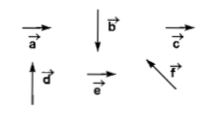Component of $3\stackrel{^}{i}+4\stackrel{^}{j}$ perpendicular to $\stackrel{^}{i}+\stackrel{^}{j}$ and in the same plane as that of $3\stackrel{^}{i}+4\stackrel{^}{j}$ is:

(1) $\frac{1}{2}\left(\stackrel{^}{j}-\stackrel{^}{i}\right)$

(2) $\frac{3}{2}\left(\stackrel{^}{j}-\stackrel{^}{i}\right)$

(3) $\frac{5}{2}\left(\stackrel{^}{j}-\stackrel{^}{i}\right)$

(4) $\frac{7}{2}\left(\stackrel{^}{j}-\stackrel{^}{i}\right)$

Concept Questions :-

Resolution of vectors
High Yielding Test Series + Question Bank - NEET 2020

Difficulty Level:

Given that  makes an angle . Which of the following options is correct?

1.

2.

3.

4.

Concept Questions :-

Resultant of Vectors
High Yielding Test Series + Question Bank - NEET 2020

Difficulty Level:

If the angle between the vector  is  $\theta$, the value of the product $\left(\stackrel{\to }{B}×\stackrel{\to }{A}\right).\stackrel{\to }{A}$ is equal to:

1.

2.

3.

4. zero

Concept Questions :-

Vector Product
High Yielding Test Series + Question Bank - NEET 2020

Difficulty Level:

Two forces A and B have a resultant ${R}_{1}$. If B is doubled, the new resultant ${R}_{2}$ is perpendicular to A. Then

(1) ${R}_{1}=A$

(2) ${R}_{1}=B$

(3) ${R}_{2}=A$

(4) ${R}_{2}=B$

Concept Questions :-

Resultant of Vectors
High Yielding Test Series + Question Bank - NEET 2020

Difficulty Level:

If  is finite then

1. $\stackrel{\to }{{u}_{1}}$ is parallel to $\stackrel{\to }{{u}_{2}}$

2. $\stackrel{\to }{{u}_{1}}$=$\stackrel{\to }{{u}_{2}}$

3. |$\stackrel{\to }{{u}_{1}}$|=|$\stackrel{\to }{{u}_{2}}$|

4. $\stackrel{\to }{{u}_{1}}$ and $\stackrel{\to }{{u}_{2}}$ are mutually perpendicular

Concept Questions :-

Scalar Product

Difficulty Level:

A body is moving under the action of two forces . Its velocity will become uniform under an additional third force $\stackrel{\to }{{\mathrm{F}}_{3}}$, given by :

1. $5\stackrel{^}{\mathrm{i}}-\stackrel{^}{\mathrm{j}}$

2. $-5\stackrel{^}{\mathrm{i}}-\stackrel{^}{\mathrm{j}}$

3. $5\stackrel{^}{\mathrm{i}}+\stackrel{^}{\mathrm{j}}$

4. $-5\stackrel{^}{\mathrm{i}}+9\stackrel{^}{\mathrm{j}}$

Concept Questions :-

Resultant of Vectors

Difficulty Level:

Two forces are such that the sum of their magnitudes is 18 N and their resultant is perpendicular to the smaller force and magnitude of resultant is 12 N. Then the magnitudes of the forces are

1. 12 N, 6 N

2. 13 N, 5N

3. 10 N, 8 N

4. 16 N, 2 N

Concept Questions :-

Resultant of Vectors
High Yielding Test Series + Question Bank - NEET 2020

Difficulty Level:

If the magnitude of sum of two vectors is equal to the magnitude of difference of the two vectors, the angle between these vectors is

1. 90°

2. 45°

3. 180°

4. 0°

Concept Questions :-

Resultant of Vectors
High Yielding Test Series + Question Bank - NEET 2020

Difficulty Level:

Six vectors  have the directions indicated in the figure. Which of the following statements may be true?(a) $\stackrel{\to }{b}+\stackrel{\to }{c}=\stackrel{\to }{-f}$                        (b) $\stackrel{\to }{d}+\stackrel{\to }{c}=\stackrel{\to }{f}$

(c) $\stackrel{\to }{d}+\stackrel{\to }{e}=\stackrel{\to }{f}$                        (d) $\stackrel{\to }{b}+\stackrel{\to }{e}=\stackrel{\to }{f}$

Concept Questions :-

Resultant of Vectors
High Yielding Test Series + Question Bank - NEET 2020

Difficulty Level:

If a vector $2\stackrel{^}{\mathrm{i}}+3\stackrel{^}{\mathrm{j}}+8\stackrel{^}{\mathrm{k}}$ is perpendicular to the vector $4\stackrel{^}{\mathrm{j}}-4\stackrel{^}{\mathrm{i}}+\mathrm{\alpha }\stackrel{^}{\mathrm{k}}$, then the value of $\mathrm{\alpha }$

1.  $-1$

2.  $-\frac{1}{2}$

3.  $\frac{1}{2}$

4.  $1$

Concept Questions :-

Scalar Product